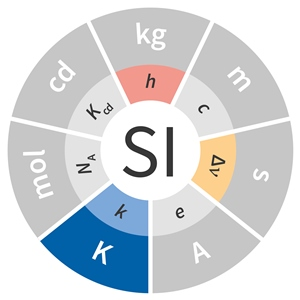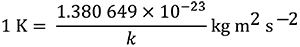# SI base unit: kelvin (K)

The kelvin, symbol K, is the SI unit of thermodynamic temperature. It is defined by taking the fixed numerical value of the Boltzmann constant k to be 1.380 649 x 10–23 when expressed in the unit J K–1, which is equal to kg m2 s–2 K–1, where the kilogram, metre and second are defined in terms of h, c and ΔνCs.This definition implies the exact relation k = 1.380 649 x 10–23 kg m2 s–2 K–1. Inverting this relation gives an exact expression for the kelvin in terms of the defining constants k, h and ΔνCs:which is equal toThe effect of this definition is that one kelvin is equal to the change of thermodynamic temperature that results in a change of thermal energy k T by 1.380 649 x 10–23 J.